Function Repository Resource:

# FindEquationalModels

Find instances of a binary operator that satisfy equational constraints

Contributed by: Stephen Wolfram
 ResourceFunction["FindEquationalModels"][eqns,f,vars] finds size-2 models of the operator f that appear in the equation eqns, with variables vars. ResourceFunction["FindEquationalModels"][eqns,f,vars,k] finds size-k models.

## Examples

### Basic Examples (4)

Find the "multiplication tables" for semigroups of size 2:

 In:=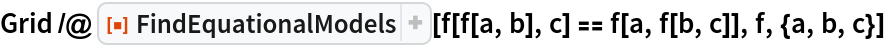Out=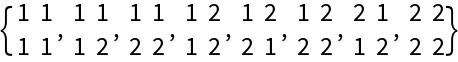Find size-3 models of an equational axiom:

 In:=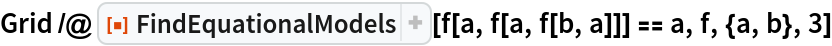Out=Find size-2 models of Wolfram's axiom:

 In:=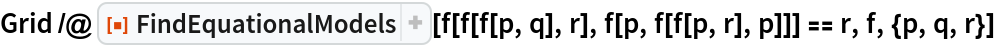Out=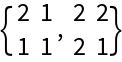There are no size-3 models:

 In:=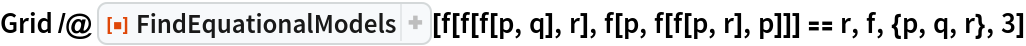Out=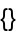## Version History

• 1.0.0 – 17 December 2019

## Author Notes

See https://www.wolframscience.com/nks/notes-12-9--implementation-of-operators-from-axioms/ which may be a better thing to do....

 In:=